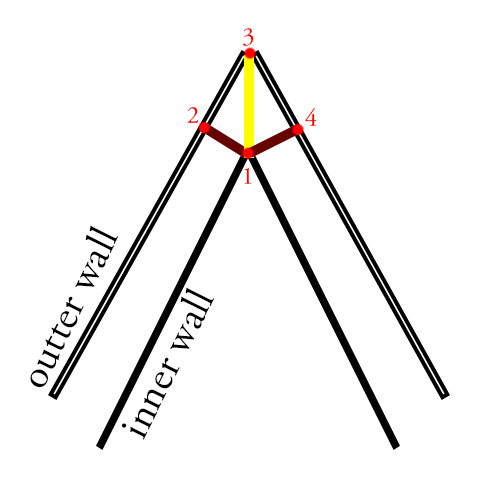# How to connect walls corners

Hello, I have no idea how to connect corners of walls. Im making walls by new mesh and stretching them to mouse position. It would be nice if I could connect walls by mesh but I dont know how to do this. Maybe someboy could help me with this. There can be many walls connected.

depends if in 2d or 3d… but possibilities…

1. just push them into each other, and let z-buffer take car of the intersections. (this still won’t be able to add extra geometry where you have sketched)
2. if your pieces always fit at pre defined angles… e.g most rooms have right angles, then build a corner piece you can use at the intersecting corners.
3. do some very complex boolean geometry intersection code, that can build new geometry. (this still won’t be able to add extra geometry where you have sketched)
4. if your images are flat (hard to tell from screen shot) you could build in a rounded end to the texture at each end… then when they overlap, the round parts would match.
5. add a nub. some kind of joint object that you put at each corner (works for 2D and 3D) a 3D wall would be a tall pillar( think of castle wall towers at angles in the wall) . for 2D it might be a Circular sprite. then the walls are stuffed into or below your nub. It hides the join, but you’ll have a nub at each joint/corner, which you might not visually want.
there are probably more ways…

Hi. I think that you could to it generating a new mesh procedurally. If your walls are always making paths made of simple polylines (no curves, just straight lines) you’ll have two scenarios: 90º corner in “L” shape, non 90º corners in “V” shape. So, in order to close the corner you’ll need 4 points (in the 2D case, in 3D you’ll need 8, four for the floor and fourfor the ceiling).

So you have the two walls meeting, each wall has the exterior and interior part (outter and inner). Then you’ll get the 4 points this way:

1. The exact point between the two walls (in the inner part)
2. If you make a line perpendicular to the inner wall segment from the point 1 to the left (+90º), then it’ll cut the outter wall segment in this point.
3. The exact point between the two walls (in the outter part)
4. If you make a line perpendicular to the inner wall segment from the point 1 to the right (-90º), then it’ll cut the outter wall segment in this point.

So your corner will be a mesh with the two triangles: 1-3-2 and 1-4-3. This will work for both cases.

If you agree that this is the right solution for your problem then I’ll also suggest that you avoid entirely intersection calculations and so on, becase I assume that you know the distance between the inner and the outter walls, therefore you can get points 2 and 4 with simple vector math which is fast and cheap in comparison with intersection math.

[161232-11111无标题.png|161232]

first sorry for bad english. if you know the direction v1, v2 ,so you can get the direction of v3 which is half angle of v1 and v2, and the cross point A, B is v3 cross v1’s two edge.

``````    Matrix4x4 GetRotationMatrixY( float radian)
{
return new Matrix4x4(
new Vector4(0, 1f, 0, 0),
new Vector4(0, 0, 0, 1f)
) ;
}

public static int LineLineIntersection2(out Vector3 intersection, Vector3 linePoint1, Vector3 lineVec1, Vector3 linePoint2, Vector3 lineVec2)
{

Vector3 lineVec3 = linePoint2 - linePoint1;
Vector3 crossVec1and2 = Vector3.Cross(lineVec1, lineVec2);
Vector3 crossVec3and2 = Vector3.Cross(lineVec3, lineVec2);

float planarFactor = Vector3.Dot(lineVec3, crossVec1and2);

//Lines are not coplanar. Take into account rounding errors.
if ((planarFactor >= 0.00001f) || (planarFactor <= -0.00001f))
{
intersection = Vector3.zero;
return -1;
}

//Note: sqrMagnitude does x*x+y*y+z*z on the input vector.
float s = Vector3.Dot(crossVec3and2, crossVec1and2) / crossVec1and2.sqrMagnitude;

intersection = linePoint1 + (lineVec1 * s);
return 0;
}

var deltaAngle = Vector3.SignedAngle(dir1, dir2, Vector3.up);//Mathf.Acos(Vector3.Dot(dir1, dir2));

var delta = deltaAngle * Mathf.Deg2Rad;
//rotate dir1
var roMat = GetRotationMatrixY(2*Mathf.PI - delta / 2f);

var newDir = roMat.MultiplyVector(dir1);
Vector3 pos ; //which is the point x

var c1 = LineLineIntersection2(out cross1, bp1, dir1, pos, newDir);
var c2 = MathLib.LineLineIntersection2(out cross2, bp2, dir1, pos, newDir);
``````